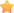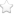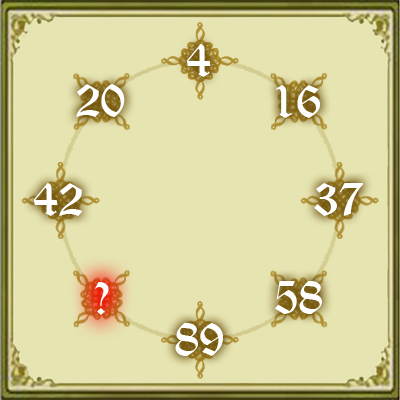×◎本題引用自遊戲學校，網友rolfuson提供

145

42 = 16
12 + 62 = 37
32 + 72 = 58
52 + 82 = 89
82 + 92 = 145
12 + 42 + 52 = 42
42 + 22 = 20
22 + 02 = 4 (一個循環)

（你有更好的解法嗎？歡迎到粉絲團遊戲學校去跟大家分享喔！）